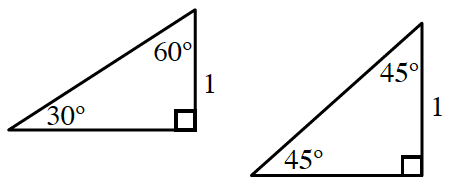### Home > INT3 > Chapter 9 > Lesson 9.1.2 > Problem9-13

9-13.Copy the triangles at right onto your paper. Homework Help ✎

1. Label the missing sides with their exact lengths. That is, leave your answers in radical form.

Refer to your work from problem 9-4 in the previous lesson.

2. A $30^\circ-60^\circ-90^\circ$ triangle is sometimes called a half-equilateral triangle. Draw a picture to illustrate this, and explain how that fact can be used to help label the missing sides in part (a).

What would happen if you changed the orientation of the triangle?

Where would the line of symmetry lie?